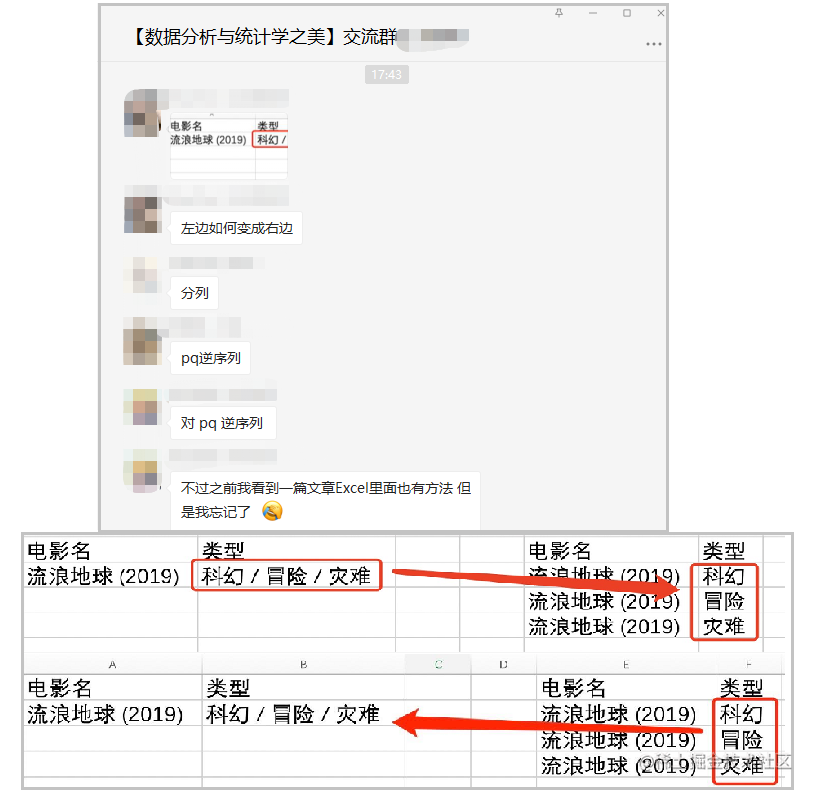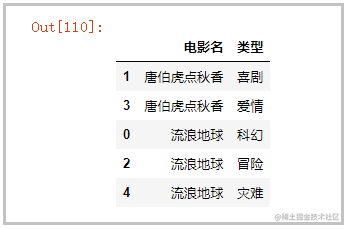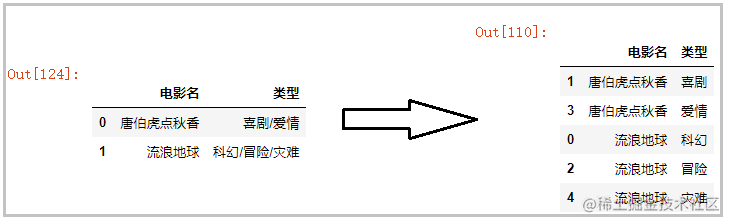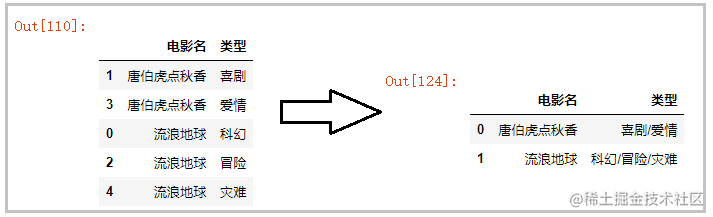# Python中实现_一行拆多行_和_多行并一行_，你会吗？## 粉丝提问## 一行拆多行

#### 1）方法一

• Pandas.melt()函数的用法；
• Series.str.split("/",expand=True)中，expand=True参数的用法；
• Series.sort_values()对文本进行排序；
• Python中enumerate()函数的用法；
``````import pandas as pd
# 读取数据
# 将一列炸裂成多列
df[["类型1","类型2","类型3"]] = df["电影类型"].str.split("/",expand=True)
# 选取想要的列
df_final = df[["电影名","类型1","类型2","类型3"]]
# 将行专列
df_final = df_final.melt(id_vars=["电影名"],value_name="类型")
# 对“电影名”字段进行排序
df_final = df_final[["电影名","类型"]]
df_final.sort_values(by="电影名",inplace=True)
# 删除“类型==None”的行
for index,value in enumerate(df_final["类型"]):
if value == None:
df_final.drop(df_final.index[index],inplace=True)
df_final#### 2）方法二

• Pandas.explode()函数的用法；
``````import pandas as pd
# 读取数据
# 将一行拆分成列表形式，注意：这里不需要使用expand=True参数
df["type"] = df["电影类型"].str.split("/")
# 直接炸裂指定列
df.explode("type")## 多行并一行

``````import pandas as pd
# 读取数据
# 分组聚合，应用某个函数
def func(df):
return ','.join(df.values)
df = df.groupby(by='电影名').agg(func).reset_index()
df